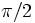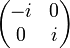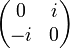# Faithful irreducible representation of dihedral group:D8

Jump to: navigation, search
This article describes a particular irreducible linear representation for the following group: dihedral group:D8. The representation is unique up to equivalence of linear representations and is irreducible, at least over its original field of definition in characteristic zero. The representation may also be definable over other characteristics by reducing the matrices modulo that characteristic, though it may behave somewhat differently in these characteristics.
For more on the linear representation theory of the group, see linear representation theory of dihedral group:D8.

## Summary

Item Value
Degree of representation 2
Schur index 1 in all characteristics
Kernel of representation trivial subgroup, i.e., it is a faithful linear representation in all characteristics except two. In characteristic two, it behaves somewhat differently. See the section #In characteristic two.
Quotient on which it descends to a faithful representation dihedral group:D8
Set of character values$\{ 2,-2,0\}$ (interpreted/reduced modulo the ring or field)
Characteristic zero: Ring generated:$\mathbb{Z}$ -- ring of integers, Ideal within ring generated:$2\mathbb{Z}$, Field generated:$\mathbb{Q}$ -- field of rational numbers
Rings of realization Realized over any unital ring, by composing the representation over$\mathbb{Z}$ with the map induced by the natural homomorphism from$\mathbb{Z}$ to the ring.
Minimal ring of realization (characteristic zero)$\mathbb{Z}$ -- ring of integers
Minimal ring of realization (characteristic$p^k$) The ring of integers mod$p^k$, i.e.,$\mathbb{Z}/p^k\mathbb{Z}$
Minimal field of realization Prime field in all cases
In characteristic zero,$\mathbb{Q}$, in characteristic$p$, the prime field$\mathbb{F}_p$
Size of equivalence class under automorphisms 1
Size of equivalence class under Galois automorphisms 1, because the representation is realized over$\mathbb{Z}$ in characteristic zero and more generally is realized over the prime subfield in any characteristic.
Bad characteristics 2, the representation can be defined but is not uniquely defined and none of the candidate representations is either faithful or irreducible. See #In characteristic two.

## Representation table

The dihedral group of order eight has a two-dimensional irreducible representation, where the element$a$ acts as a rotation (by an angle of$\pi/2$), and the element$x$ acts as a reflection about the first axis. The matrices are:$a \mapsto \begin{pmatrix}0 & -1 \\ 1 & 0 \\\end{pmatrix}, \qquad x \mapsto \begin{pmatrix}1 & 0 \\ 0 & -1 \\\end{pmatrix}.$

This particular choice of matrices give a representation as orthogonal matrices, and in fact, the representation is as signed permutation matrices (i.e., it takes values in the signed symmetric group of degree two). Thus, it is also a monomial representation.

Below is a description of the matrices based on the above choice as well as another formulation involving complex unitary matrices:

Element Matrix (orthogonal/monomial/signed permutation matrices) Matrix as complex unitary Characteristic polynomial Minimal polynomial Trace, character value Determinant$e$$\begin{pmatrix} 1 & 0 \\ 0 & 1 \\\end{pmatrix}$$\begin{pmatrix} 1 & 0 \\ 0 & 1 \\\end{pmatrix}$$(t - 1)^2 = t^2 - 2t + 1$$t - 1$ 2 1$a$$\begin{pmatrix}0 & -1 \\ 1 & 0 \\\end{pmatrix}$$\begin{pmatrix}i & 0 \\ 0 & -i \\\end{pmatrix}$$t^2 + 1$$t^2 + 1$ 0 1$a^2$$\begin{pmatrix} -1 & 0 \\ 0 & -1 \\\end{pmatrix}$$\begin{pmatrix} -1 & 0 \\ 0 & -1 \\\end{pmatrix}$$(t + 1)^2 = t^2 + 2t + 1$$t + 1$ -2 1$a^3$$\begin{pmatrix}0 & 1 \\ -1 & 0 \\\end{pmatrix}$$\begin{pmatrix}-i & 0 \\ 0 & i \\\end{pmatrix}$$t^2 + 1$$t^2 + 1$ 0 1$x$$\begin{pmatrix}1 & 0 \\ 0 & -1 \\\end{pmatrix}$$\begin{pmatrix} 0 & 1 \\ 1 & 0 \\\end{pmatrix}$$t^2 - 1$$t^2 - 1$ 0 -1$ax$$\begin{pmatrix}0 & 1 \\ 1 & 0 \\\end{pmatrix}$$\begin{pmatrix} 0 & i \\ -i & 0 \\\end{pmatrix}$$t^2 - 1$$t^2 - 1$ 0 -1$a^2x$$\begin{pmatrix}-1 & 0 \\ 0 & 1 \\\end{pmatrix}$$\begin{pmatrix} 0 & -1 \\ -1 & 0 \\\end{pmatrix}$$t^2 - 1$$t^2 - 1$ 0 -1$a^3x$$\begin{pmatrix}0 & -1 \\ -1 & 0 \\\end{pmatrix}$$\begin{pmatrix} 0 & -i \\ i & 0 \\\end{pmatrix}$$t^2 - 1$$t^2 - 1$ 0 -1
Set of values used$\{ 0,1,-1 \}$$\{ 0,1,-1,i,-i \}$ -- --$\{ 2,-2,0 \}$$\{ 1,-1 \}$
Ring generated by values used (characteristic zero)$\mathbb{Z}$ -- ring of integers$\mathbb{Z}[i]$ -- ring of Gaussian integers -- --$\mathbb{Z}$ -- ring of integers$\mathbb{Z}$ -- ring of integers
Field generated by values used (characteristic zero)$\mathbb{Q}$ -- field of rational numbers$\mathbb{Q}(i) = \mathbb{Q}[t]/(t^2 + 1)$ -- --$\mathbb{Q}$ -- field of rational numbers$\mathbb{Q}$ -- field of rational numbers

## Character

FACTS TO CHECK AGAINST (for characters of irreducible linear representations over a splitting field):
Orthogonality relations: Character orthogonality theorem | Column orthogonality theorem
Separation results (basically says rows independent, columns independent): Splitting implies characters form a basis for space of class functions|Character determines representation in characteristic zero
Numerical facts: Characters are cyclotomic integers | Size-degree-weighted characters are algebraic integers
Character value facts: Irreducible character of degree greater than one takes value zero on some conjugacy class| Conjugacy class of more than average size has character value zero for some irreducible character | Zero-or-scalar lemma

### Character values and interpretations

The character can be computed using any of the interpretations provided. See below:

Conjugacy class Size of conjugacy class Character value Size-degree-weighted character value Interpretation as dihedral group (value =$2\cos(2\pi k/n)$ for$a^k$, 0 for reflection) Interpretation as half of the induced class function from the sign representation of the center (see Induced class function from conjugacy-closed normal subgroup is index of subgroup times class function inside the subgroup and zero outside the subgroup) Philosophical justification$\{ e \}$ 1 2 1$2\cos(0) = 2(1) = 2$$(1/2)4 = 2$ character value at identity element equals degree$\{ a^2 \}$ 1 -2 -1$2\cos((2\pi)2/4) = 2\cos\pi = 2(-1) = -2$$(1/2)(-4) = -2$ central, so must go to a central element. Since the representation is faithful, must not go to the identity, so must go to a non-identity scalar matrix of order 2. Only possibility is scalar matrix of$-1$s.$\{ a, a^3 \}$ 2 0 0$2\cos(2\pi/4) = 2\cos(\pi/2) = 2(0) = 0$ 0 (outside the center) conjugacy class of more than average size has character value zero for some irreducible character -- and its value is nonzero on all the other characters, because they're one-dimensional.$\{ x, a^2x \}$ 2 0 0 0 since reflection 0 (outside the center) conjugacy class of more than average size has character value zero for some irreducible character -- and its value is nonzero on all the other characters, because they're one-dimensional.$\{ ax, a^3x \}$ 2 0 0 0 since reflection 0 (outside the center) conjugacy class of more than average size has character value zero for some irreducible character -- and its value is nonzero on all the other characters, because they're one-dimensional.

## In characteristic two

Field of characteristic not two case Explanation Field of characteristic two case Explanation You were wondering ...
There are many different forms of the representation, all equivalent as linear representations We see from the table that the representations all have the same character, and character determines representation when the characteristic of the field does not divide the order of the group. So, any two such representations are equivalent. There are multiple different forms of the representation, and they are not equivalent as linear representations. For instance, the representation as monomial matrices in the table above, when reduced mod 2, gives a representation with kernel one of the Klein four-subgroups of dihedral group:D8. If we pre-compose the representation with the automorphism$a \mapsto a, x \mapsto ax$, we get an equivalent representation in characteristic zero. However, in characteristic two, the new representation has a different kernel -- the other Klein four-subgroup -- and is therefore not equivalent. Why doesn't the conjugating matrix that establishes the equivalence of representations in characteristic zero reduce to a matrix in characteristic two that establishes the equivalence of representations? The key is that the matrix (and its inverse) establishing equivalence of representations has entries in$\mathbb{Q}$ and not all in$\mathbb{Z}$, and it cannot be reduced modulo 2. More explicitly, some of the matrix entries have denominators divisible by 2 and hence the matrices cannot be interpreted modulo 2.
All representations are faithful. None of the elements other than the identity maps to the identity matrix, regardless of the choice of$\alpha, \beta$. None of the representations are faithful. Since$1 = -1$ in characteristic two, the elements$\{ e, a^2\}$ of the dihedral group both map to the identity matrix. Hence, the kernel contains the center of dihedral group:D8 and the representation is not faithful. If we use the specific form of matrices indicated above, the kernel is one of the Klein four-subgroups, which is even bigger.
All representations are irreducible. Can be verified by computing the character norm, or by noting that there is no invariant one-dimensional subspace. All representations are indecomposable but not irreducible. Follows from general facts about action on$p$-groups: the fixed-point subspace must be nonzero and is hence an invariant one-dimensional subspace.

## Relation with faithful linear representations

In a field of characteristic not equal to two, a linear representation of the dihedral group of order eight is faithful if and only if the faithful irreducible representation (that forms the subject of this page) occurs as one of its irreducible subrepresentations (i.e., one of its summands in its direct sum decomposition by Maschke's theorem) with multiplicity one or higher. We note both directions:

• Faithful irreducible representation as a subrepresentation implies faithful: The kernel of any subrepresentation contains the kernel of the whole representation, so this follows.
• Faithful implies contains the faithful irreducible representation as a subrepresentation: The dihedral group of order eight has only one faithful irreducible representation. Moreover, for all other irreducible representations, the kernel of the representation contains the center of dihedral group:D8. Thus, any representation that is a direct sum of these representations has nontrivial kernel (at least the center of dihedral group:D8) and cannot be faithful.

## Embeddings

Since this representation makes sense over finite fields, and it is faithful if the characteristic is not two, it provides an embedding of dihedral group:D8 in the general linear group of degree two over any finite field of characteristic not two. In fact, the representation goes to the orthogonal group for the standard dot product, which is one of the two possible orthogonal groups for the finite field (which one it is depends on the congruence class of the size mod 4).

Further, since this is a unique faithful representation of degree two, the embedding is as isomorph-conjugate subgroups inside the general linear group.

Field size$q$ Field information General linear group of degree two Orthogonal group Embedding of dihedral group:D8 in general linear group of degree two Embedding of dihedral group:D8 in orthogonal group
3 field:F3 general linear group:GL(2,3)  ? D8 in GL(2,3)  ?
5 field:F5 general linear group:GL(2,5)  ? D8 in GL(2,5)  ?

## Semidirect products

For any linear representation, we can construct an external semidirect product with normal subgroup the additive group of the vector space and acting quotient the group being represented. We describe below some of the semidirect products in the case of finite fields.

PLACEHOLDER FOR INFORMATION TO BE FILLED IN: [SHOW MORE]

## GAP implementation

### Construction of representation as a homomorphism

The representation over characteristic zero can be constructed using:

gap> G := DihedralGroup(8);; phi := Filtered(IrreducibleRepresentations(G),x -> Length(Identity(G)^x)= 2);
Pcgs([ f1, f2, f3 ]) -> [ [ [ 0, 1 ], [ 1, 0 ] ], [ [ E(4), 0 ], [ 0, -E(4) ] ], [ [ -1, 0 ], [ 0, -1 ] ] ]

The set of images of all elements can be computed as matrices. For instance, once the representation is defined as above, the following displays the elements and their matrix images in paired form:

gap> List(Elements(G),x -> [x,x^phi]);
[ [ <identity> of ..., [ [ 1, 0 ], [ 0, 1 ] ] ],
[ f1, [ [ 0, 1 ], [ 1, 0 ] ] ], [ f2, [ [ E(4), 0 ], [ 0, -E(4) ] ] ],
[ f3, [ [ -1, 0 ], [ 0, -1 ] ] ], [ f1*f2, [ [ 0, -E(4) ], [ E(4), 0 ] ] ],
[ f1*f3, [ [ 0, -1 ], [ -1, 0 ] ] ],
[ f2*f3, [ [ -E(4), 0 ], [ 0, E(4) ] ] ],
[ f1*f2*f3, [ [ 0, E(4) ], [ -E(4), 0 ] ] ] ]

We can do a similar construction over other fields. For instance, over field:F3, we need to do:

gap> G := DihedralGroup(8);; phi := Filtered(IrreducibleRepresentations(G,GF(3)),x -> Length(Identity(G)^x)= 2);
Pcgs([ f1, f2, f3 ]) -> [ [ [ 0, 1 ], [ 1, 0 ] ], [ [ E(4), 0 ], [ 0, -E(4) ] ], [ [ -1, 0 ], [ 0, -1 ] ] ]

### Construction of character

The character can be constructed by searching for the appropriate row in the character table:

gap> C := Filtered(Irr(CharacterTable(DihedralGroup(8))),x -> DegreeOfCharacter(x) = 2);
Character( CharacterTable( <pc group of size 8 with 3 generators> ), [ 2, 0, 0, -2, 0 ] )